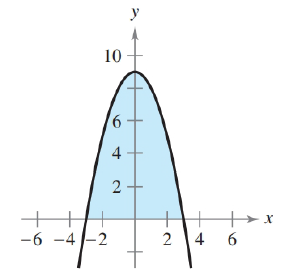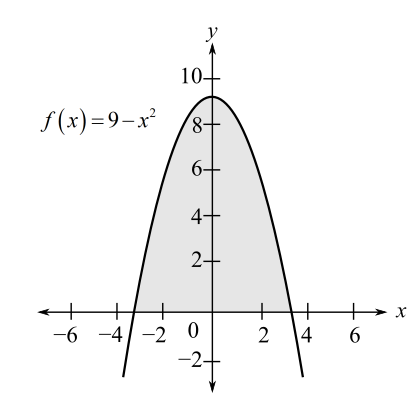Chapter 5, Problem 54RE### Calculus: An Applied Approach (Min...

10th Edition
Ron Larson
ISBN: 9781305860919

#### Solutions

Chapter
Section### Calculus: An Applied Approach (Min...

10th Edition
Ron Larson
ISBN: 9781305860919
Textbook Problem
1 views

# Finding Area by the Fundamental Theorem In Exercises 53-58, find the area of the region. f ( x ) = 9 − x 2To determine

To calculate: The area of region bounded by graph of function f(x)=9x2Explanation

Given Information:

The provided graph of function f(x)=9x2.

Formula used:

Area of function f(x) bound from x=a and x=b is given by

Area=abf(x)dx

The fundamental theorem of calculus:

abf(x)dx=F(b)F(a)

Here, F is function such that F(x)=f(x) for all x in [a,b].

Calculation:

The provided graph of function f(x)=9x2.

The graph of function f(x)=9x2 is parabola bounded from x=3 and x=3. The integration of function f(x)=9x2 from x=3 and x=3 represent the area of region bound graph

### Still sussing out bartleby?

Check out a sample textbook solution.

See a sample solution

#### The Solution to Your Study Problems

Bartleby provides explanations to thousands of textbook problems written by our experts, many with advanced degrees!

Get Started

#### Find more solutions based on key concepts